# Ray tracing the Next Week - Cornell Box

I'm so excited to learn ray tracing and did a lot of fun. But I'm stuck at rendering empty Cornell Box scene.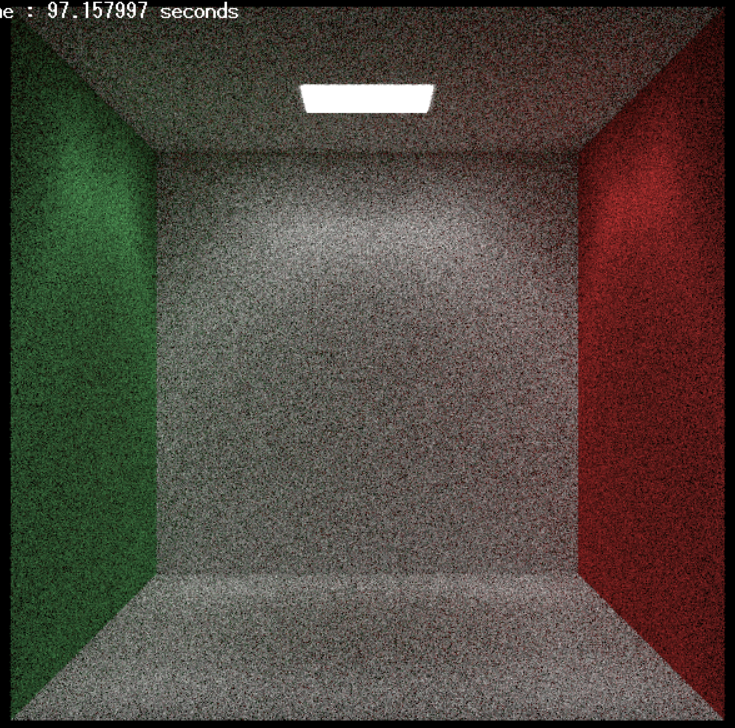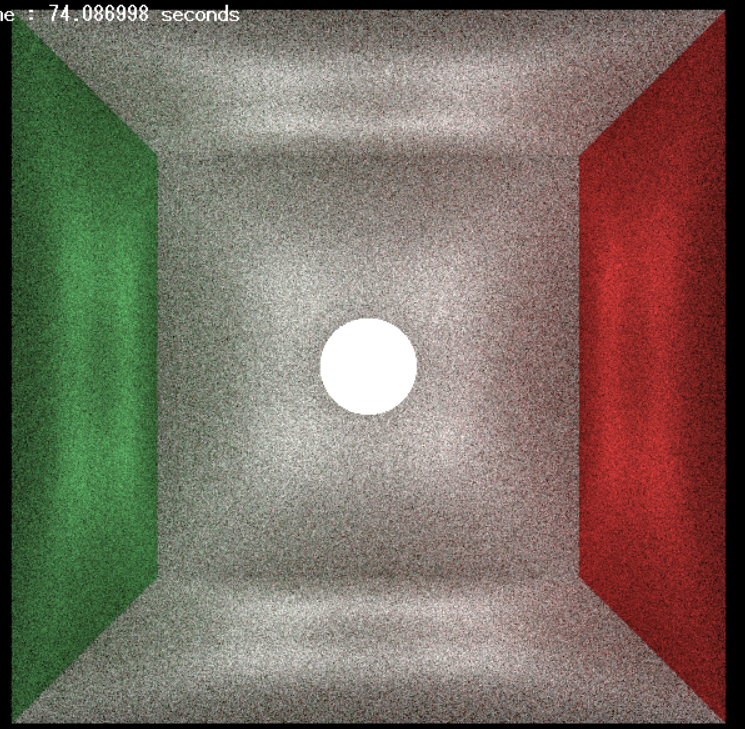It has some darker color along the bright color and it's seem different with the image in the book. Sorry if my English confuses you because I'm still learning it.

• Hi, welcome to GDSE! Please post your solution as an answer to the question, instead of editing it into the question. This way others can find help more easily in the future, as they can see that the question has an answer! Feb 3 at 9:37
• @Tyyppi_77 thanks for remine me. I just edit my answer. This is first time I post so I don't have experiment. Feb 3 at 11:42
• For future reference, you might want to ask questions like this on the Computer Graphics StackExchange instead. Uses of raytracing and path tracing in games are still an emerging field, but better established in offline rendering. Feb 3 at 18:43
• @DMGregory Great site for discussing about Graphics Programming. Thanks for suggestion. Feb 4 at 1:16

I solved this.

The bright color is look like rectangle because the light that is reflected by Lambertian material is scattered randomly in unit cube (with center is the unit normal) . I fixed this by just allow ray is scattered randomly point in unit sphere.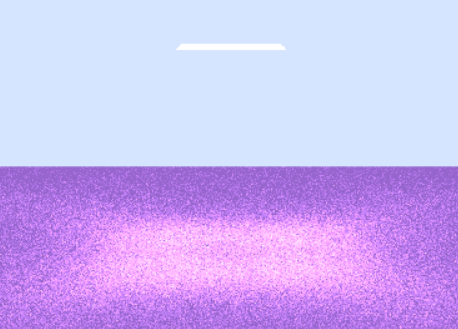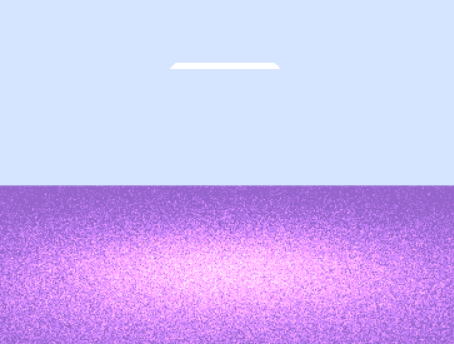Final result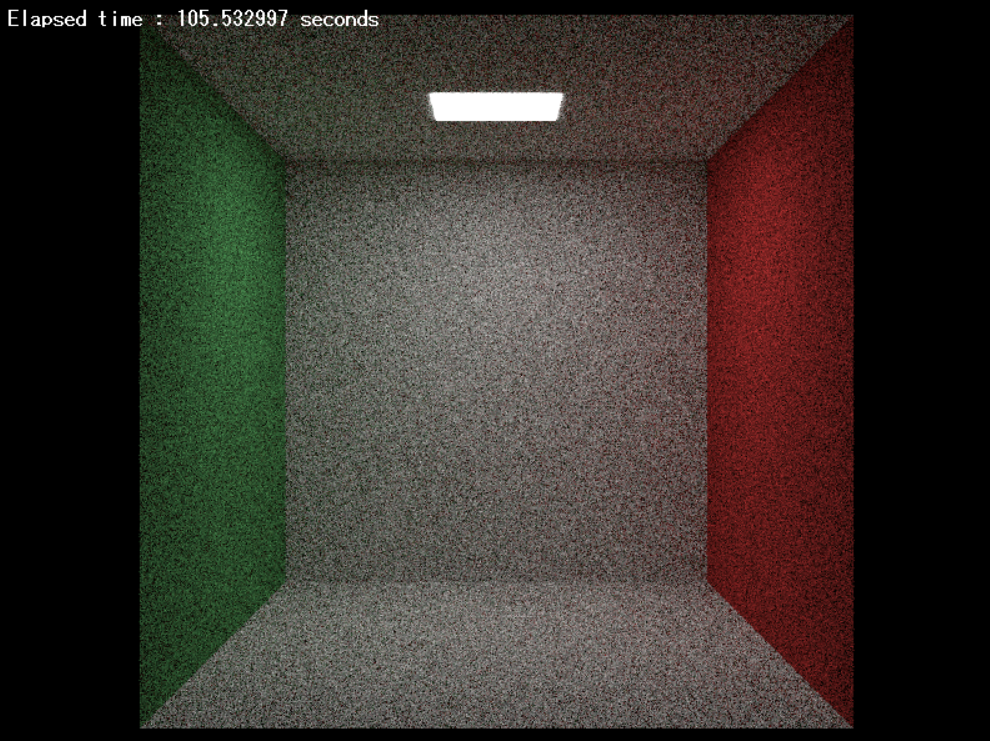Example code :

Function to generate random vector

vec3 random(float min, float max)
{
return { Random(min, max), Random(min, max), Random(min, max) };
}


Code show generate random position in unit sphere, with using dot product to check the the vector is in radius of unit sphere, and reject it when vector is generated outside the unit sphere.

vec3 random_in_unit_sphere()
{
vec3 v = random(-1.0f, 1.0f);
while (dot(v, v) >= 1)
v = random(-1.0f, 1.0f);
return v;
}


Then from the hit point we just use normal vector of hit surface like the center of unit sphere, and add with random vector position in unit sphere. This random vector will work as direction vector that bend normal vector to direction of scatter ray.

scatter_ray.direction = hit_surface.normal + random_in_unit_sphere();

• Can you show the code you changed to make the scattering happen in a sphere instead of a cube? Feb 3 at 12:12
• @DMGregory I just add my code as expend answer. Sorry for my bad English. Every things I learned is from Ray tracing series github.com/RayTracing/raytracing.github.io. Feb 3 at 13:12